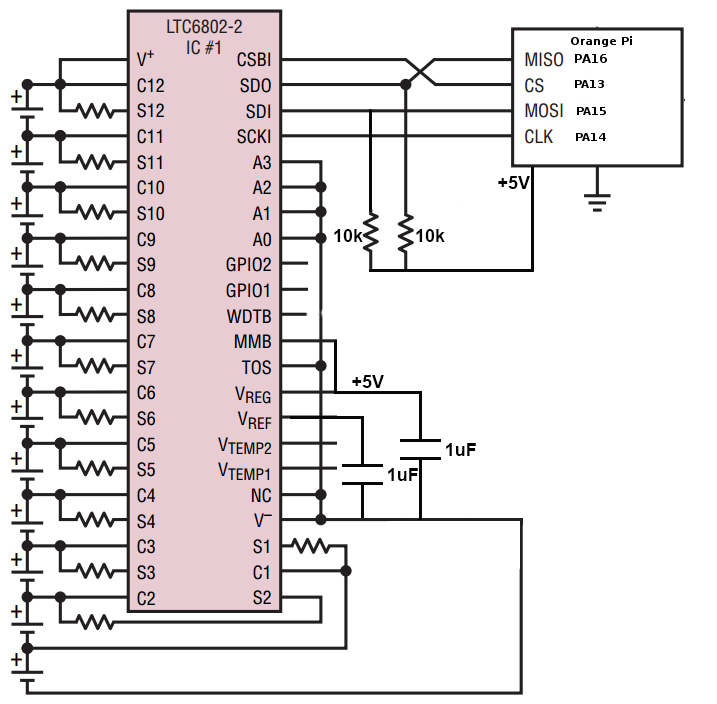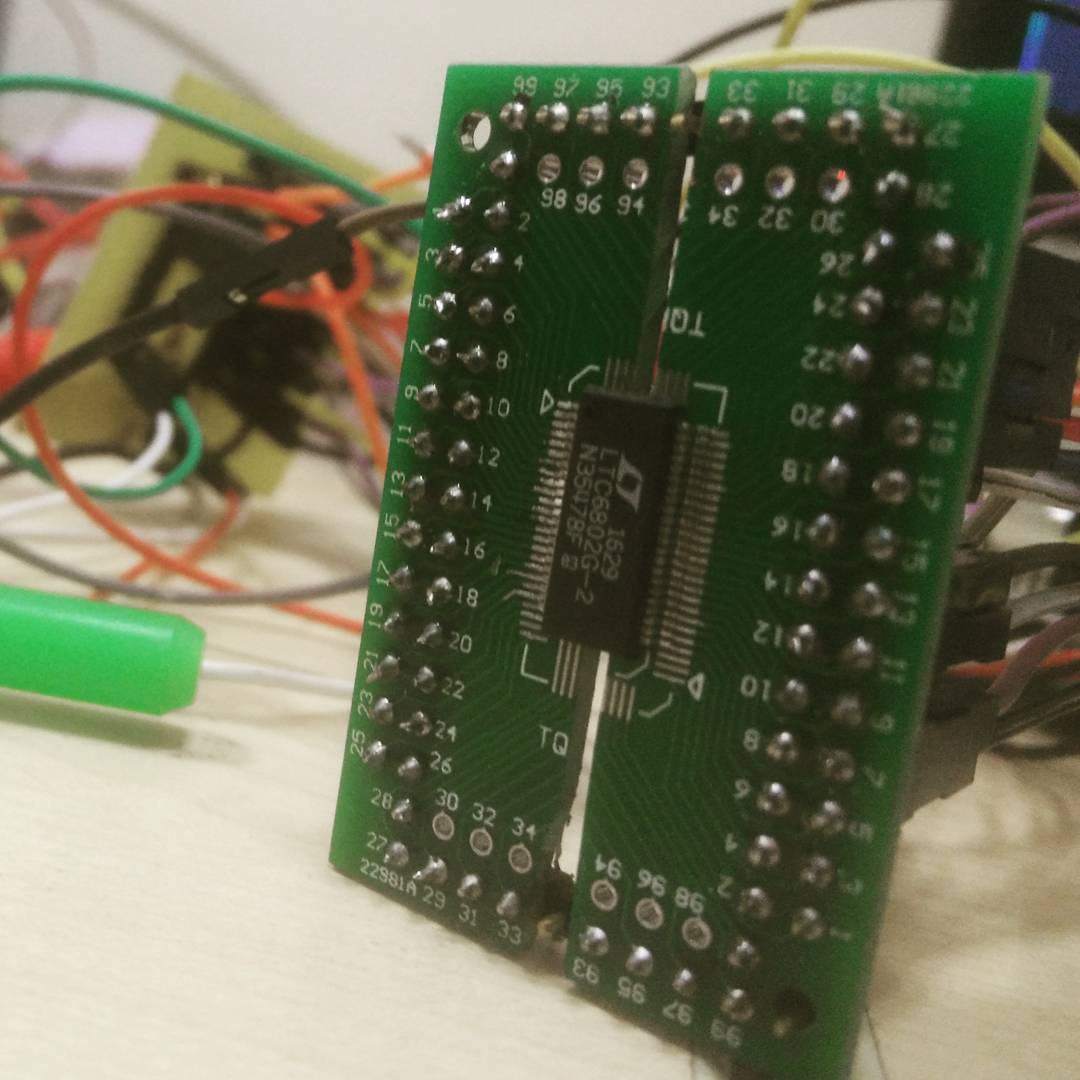# LTC6802

The LTC6802 is a complete battery monitoring IC that includes a 12-bit ADC, a precision voltage reference, a high voltage input multiplexer and a serial interface. Each LTC6802-2 can measure up to 12 series connected bat-tery cells with an input common mode voltage up to 60V.

LTC6802 is a great option if you want to build battry monitoring system with more accuracy. This IC operates on SPI protocol.

The datasheet for LTC6802-2 can be found at here

Following figure shows the circuit diagram to interface the LTC6802-2 with Orange Pi zero.After mounting the IC on PCB it looks like this.# Interfacing with Orange-Pi

In order to access the data from IC over SPI, I’m using periphery.

to install periphery:

``\$ pip install python-periphery``

To activate the LTC chip we need to make the CS pin low. To do that I’m using pyA20 library for Orange Pi.

to install pyA20:

``\$ pip install pyA20``

The standard procedure to access the data from LTC6802 over SPI is:

• make CS pin low.

• write the data/command to SPI.

• read the output from the SPI.

• make CS pin high.

Since the circuit and the dependencies are in place, let’s start coding.

``````#!/usr/bin/env python
# -*- coding: utf-8 -*-

from periphery import SPI
from pyA20.gpio import gpio as io
from pyA20.gpio import port
import time

cs  = port.PA13                         # Chip select pin
spi = SPI("/dev/spidev1.0", 0, 1000000) # Spi port

io.init()                 # init GPIO
io.setcfg(cs, io.OUTPUT)  # configure cs Pin as output pin.

RDCV    = 0x04   # Read cells
STCVAD  = 0x10   # Start all A/D's - poll status

address = 0x80   # as we are connecting only one IC the address is 0x80
# refer the datasheet for the details about addressing of LTC6802

# refer datasheet for more details about configuration.
Config = [0x01, 0x01, 0x00, 0x00, 0x00, 0x71, 0xAB]

def writeCfg():

"""
Writes the configuration to IC.
"""
io.output(cs, io.LOW)            # make CS low
data_in = spi.transfer(Config)   # write the config to IC and read the OP
io.output(cs, io.HIGH)           # make CS high

def readVoltage():

"""
Returns a dictionary containing the voltages of 12 battries.
"""

v = {}
io.output(cs, io.LOW)            # make CS low
data_out = [STCVAD]
data_in = spi.transfer(data_out) # start all A/D
time.sleep(0.02)
io.output(cs, io.HIGH)           # make CS high
io.output(cs, io.LOW)            # again make CS low
spi.transfer([0x80])             # write the address 0x80 to SPI
spi.transfer([RDCV])             # (command to) read cells

data = []

for i in range(18):                   # read 18 bytes from the IC
data.append(spi.transfer([RDCV]))
io.output(cs, io.HIGH)

# refer the datasheet of LTC6802 for conversion of ADC value to Voltages.

# here we are calculating voltages from ADC values of all 12 battries.

v['0']  = ((data & 0xFF) | (data & 0x0F) << 8)*1.5*0.001
v['1']  = ((data & 0xF0) >> 4 | (data & 0xFF) << 4)*1.5*0.001
v['2']  = ((data & 0xFF) | (data & 0x0F) << 8)*1.5*0.001
v['3']  = ((data & 0xF0) >> 4 | (data & 0xFF) << 4)*1.5*0.001

v['4']  = ((data & 0xFF) | (data & 0x0F) << 8)*1.5*0.001
v['5']  = ((data & 0xF0) >> 4 | (data & 0xFF) << 4)*1.5*0.001
v['6']  = ((data & 0xFF) | (data & 0x0F) << 8)*1.5*0.001
v['7']  = ((data & 0xF0) >> 4 | (data & 0xFF) << 4)*1.5*0.001

v['8']  = ((data & 0xFF) | (data & 0x0F) << 8)*1.5*0.001
v['9'] = ((data & 0xF0) >> 4 | (data & 0xFF) << 4)*1.5*0.001
v['10'] = ((data & 0xFF) | (data & 0x0F) << 8)*1.5*0.001
v['11'] = ((data & 0xF0) >> 4 | (data & 0xFF) << 4)*1.5*0.001

return v

def readVal():

while True:
writeCfg()
time.sleep(0.001)
v = readVoltage()
print(v)
time.sleep(1)

if __name__ == '__main__':

readVal()
``````
``````The minimum operating voltage of LTC6802 is 10V.
Make sure that total voltage of all the battries combined together is more than 10V.``````